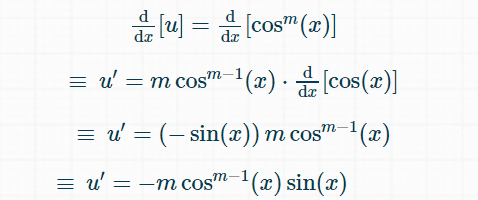# Integration Problem

## Homework Statement

Derive that:Correction (from a later post)
The integral on the left side should be ##\int \cos^m(x)\sin^n(x)~dx##

-

## The Attempt at a Solution

Anyone can help me on how to start?

## u = cos^m x ##

## du = m cos^{m-1} x ##

## dv = sin^n x dx ##

## v = \frac{sin^{n+1} x}{n} ##

#### Attachments

Last edited by a moderator:

BvU
Homework Helper
Hi,
##du = m \cos^{m-1} x## can not be correct; the left hand side is a differential and the right hand side is not. Also, it is clearly wrong for ##m = 1## ....

Hi,
##du = m \cos^{m-1} x## can not be correct; the left hand side is a differential and the right hand side is not. Also, it is clearly wrong for ##m = 1## ....
How to derive ## u = cos^{m} \,\,\, x ## ?

Last edited:
BvU
Homework Helper
You use the chain ruleYou use the chain rule#### Attachments

•BvU
BvU
Homework Helper
Now that we have settled that: is there a typo in the problem statement ? On the left I see no variable ##n## !?

Now that we have settled that: is there a typo in the problem statement ? On the left I see no variable ##n## !?
yeah , it should be ∫cosmx sinndx

BvU
Homework Helper
Doesn't look like the expression in post #1 yet, does it ?
What could be the trick to get e.g. ##m+n## in the denominator ?

How can I ?

Ray Vickson
Homework Helper
Dearly Missed
How can I ?

You cannot. Your are being asked to show something that is false. If you let ##L## be your (corrected) left-hand-side and ##R## the right-hand-side, then trying out some small integer values of ##m## and ##n## will show that the result is wrong. For example:
$$\begin{array}{cl} m=2,n=2: & L = -\frac{1}{4} \cos(x)^3 \sin(x)+ \frac{1}{8} \cos(x) \sin(x)+ \frac{1}{8} x&\\ & R = \frac{1}{4} \cos(x) \sin(x)^3- \frac{1}{8} \cos(x) \sin(x)+\frac{1}{8} x \\ m=5,n=3: & L = - \frac{1}{8} \cos(x)^6 \sin(x)^2- \frac{1}{24} \cos(x)^6 \\ &R = \frac{1}{8} \cos(x)^4 \sin(x)^4-\frac{1}{10} \cos(x)^3 \sin(x)^2- \frac{1}{15} \cos(x)^3 \end{array}$$

You can check these using a computer algebra system; I used Maple, but you can do it (at no cost) using Wolfram Alpha, for example.

Last edited:
Mark44
Mentor
Checking some online tables of trig integrals, I found this one that is nearly the same as that in the OP:

$$\int \cos^m(x)\sin^n(x)dx = \frac{\cos^{m-1}(x)\sin^{n+1}(x)}{m + n} + \frac{m - 1}{m + n}\int \cos^{m-2}(x)\sin^n(x)dx$$
The main difference between this integral formula and the one in post #1 is the exponent on the cosine factor in the last integral.
In post 1, the exponent is m - n. In the formulat just above, the exponent is m - 2. As @Ray Vickson points out, the integral as posted isn't correct, so it's very likely that the m-n exponent is a typo.